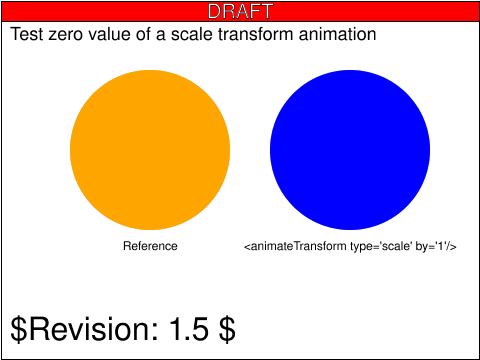Tests that contain the draft-watermark are under development and may be incorrectly testing a feature.

# animate-elem-87-t.svg## Operator Script

The test consists of two circles: an orange circle on the left, which serves as a reference, and a blue circle on the right, whose `transform` attribute is animated. Animation of the circles begins at t=1s and lasts for 3s.

The transform animation that applies to the blue circle is of type "scale", and specifies `by="1"`. Since the animation is considered to be equivalent to one that specifies `from="0" to="1"`, when the animation begins the circle will be scaled down to a point, and then will be scaled up until it reaches its original size at t=4s.

## Pass Criteria

The test is passed if the blue circle on the right is always the same size as the orange circle on the left. The test runs from t=0s until t=4s.

## Test Description

This tests that the underlying value of a scale transformation is 0. Since SMIL defines a by animation as being equivalent to an additive values animation where the first value is zero and the second value is the by value, such an animation would begin by post-multiplying a `scale(0)` transformation to the element's underlying transform list.